# Introduction

In the previous article, we discussed Neural Turing Machines(NTMs), which introduced an external memory to maintain information for later retrieval. In this post, we further discuss a more sophisticated method, namely Differential Neural Computer(DNC). The DNC builds on the same idea as the NTMs — both aim to combine the advantage of neural and computational processing by providing a neural network with read-write access to external memory. On the other hand, it improves NTMs with a more complicated and flexible interaction mechanism, which makes it potentially more powerful than NTMs.

# Overview of Differential Neural Computer

The above figure from the original paper demonstrates the overall architecture of a DNC. The following figure further details its internal structure.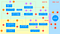The internal structure of a DNC. Symbols in purple squares are from the interface vector; those in orange squares represent the previous states of DNC; those in red squares denote the current states. Operations are defined in the blue rectangles

We first provide a brief overview of each part in the DNC cell, a detailed discussion will come shortly.

• The controller(generally an LSTM cell) receives inputs from the environment together with previous read vectors and emits an output vector and an interface vector, the latter of which is responsible for the interaction with the write and read module.
• The write module manipulates the memory matrix by integrating the content-based look-up and allocation mechanism. The former generates a weighting by measuring the similarity between the input write key and memory entries, while the latter generates weighting based on the usages of each memory location.
• The read module weights three types of memories: content-based memory instructed by the read keys, and temporal memory in the forward and backward written order. We address the first type of memory using the content-based look-up and the rest using a temporal linking mechanism, which leverages a form of a learnable adjacent matrix to track the sequential order.

# Controller Network

We summarize the controller into three steps

1. At every time-step t the controller network receives input vector x_t∈R^X from the environment(or a dataset) and a set of R read vectors _{t-1}, …, r_{t-1}^R from the memory matrix M_{t-1} ∈ R^{N⨉W} at the previous time-step, via the read heads.

2. It then concatenates the read and input vectors to obtain a single controller input vector X_t=[x_t;r_{t-1}¹; …, r_{t-1}^R] and pass it through a deep LSTM architecture: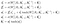l is the layer index, 𝜎(x) is the logistic sigmoid function, h_t^l, i_t^l, f_t^l, s_t^l, and o_t^l are the hidden, input gate, forget gate, (cell)state and output gate activation vectors, respectively, of layer l at time t; h⁰_t=0 for all t; h_0^l=s_0^l=0 for all l. These are just components of a regular LSTM stack; there is nothing new here.

3. The controller emits an output vector ​v_t, defined as

The output vector v_t​ is then combined with the current read vectors to produce the final output — action distribution for reinforcement learning, or predictive distribution for supervised learning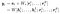in the second step, we combine W_y and W_r into a single layer W

This arrangement allows the DNC to condition its output dimensions on memory that has just been read.

The controller also produces an interface vector 𝜉_t∈R^{(W\times R)+3W+5R+3}, defined as

The interface vector encodes its interaction with memory at the current time step, similar to NTMs discussed in the previous post, but using different mechanisms to build read and write weightings.

## Interface Parameters

Before we describe how the interface vector 𝜉_t​ interacts with the external memory of shape ​N⨉W, we subdivide it as follows:

The components with ^ are then processed with various functions to ensure that they lie in the correct domain. After that we have the following set of scalars and vectors:

where the oneplus function and unit simplex S_N​ are defined as follows:

we further introduce a weighting space over N​ locations, which only differs from unit simplex S_N​ in that 𝛼​ does not necessarily sum to one.

## A Quick View of Reading and Writing

We delay the computation of reading and writing weightings w_t^{r,1},…,w_t^{r,R},w_t^w to the next sub-section. For now, we assume these weightings have been computed. The read and write vectors are computed in the same way as they are in NTMs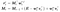∘ denotes element-wise multiplication and E is an N⨉W matrix of ones.

Noticeably, the weightings used above do not necessarily sum to one.

As we stated before, the system uses a combination of content-based addressing and dynamic memory allocation to determine where to write in memory, and a combination of content-based addressing and temporal memory linkage to determine where to read. These mechanisms, all of which are parameterized by the interface vectors defined in section ‘Interface Parameters’, are described below.

As NTMs, read and write heads use content-based addressing for associative recall and modifying an existing vector in memory, respectively. Importantly, this enables a key that only partially matches the content of a memory location still to be used to attend strongly to that location. Accordingly, it allows a form of pattern completion whereby the value recovered by reading the memory location includes additional information that is not present in the key.

We compute the content weighting through a softmax function with an amplified cosine similarity

where k and 𝛽​ are the read/write key and read/write strength, respectively. We could vectorize this computation as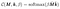\hat M is M with l2-normalized rows and \hat k is l2-normalized k.

## Dynamic Memory Allocation

To allow the controller to free and allocate memory as needed, Graves&Wayne et al. developed a differentiable analog of the ‘free list’ memory allocation scheme, whereby a list of available memory locations is maintained by adding to and removing addresses from a linked list.

1. The controller emits a set of the free gates f_t^i, one per reading head, that determine whether the most recently read locations can be freed.

2. We compute from the free gates the memory retention vector 𝜓_t ∈[0,1]^N, which represents how much each location will not be freed by the free gates:

3. The memory usage vector can then be defined as

with u_0=0 and u_t[0,1]^N. Intuitively, locations are used if they have been retained by the free gates (𝜓_t[i]≈1), and either were already in use(u_{t-1}1) or have had just been written to(w_{t-1}^w1). The usage can only be subsequently decreased using the free gates(since the content in the bracket is always greater than or equal to u_{t-1} given that w_{t-1}^w≥0 — it equals to u_{t-1} only when w_{t-1}^w=0. One could also take the part inside bracket as a set union operation, where u_{t-1} and w_{t-1}^w are two sets and u_{t-1}w_{t-1}^w is their intersection.

4. Once u_t has been determined, the free list 𝜙_t∈Z^N is defined by sorting the indices of the memory locations in ascending order of usage.

5. The allocation weighting a_t∈ 𝛥_N, which is used to provide new locations for writing, is defined as

Intuitively, the allocation weighting a_t[𝜙_t[j]] is in direct proportion to usages of locations that have more available space than 𝜙_t[j] — the more usages of these locations are used, the more memory at 𝜙_t[j] is allocated — and in inverse proportion to usage of 𝜙_t[j] itself. The former guarantees always filling locations with more available space.

As noted by the authors, the sort operation in step 4 induces discontinuities at the points at which the sort order changes. We ignore these discontinuities when calculating the gradient, as they do not seem to be relevant to learning.

Noticeably, the allocation mechanism is independent of the memory size(in fact, all elements in the interface vector are), meaning that DNCs can be trained to solve a task using one size of memory and later upgraded to a larger memory without retraining.

## Write Weighting

Now we combine the content-based addressing and dynamic memory allocation to form the write weighting. We use the memory from the previous time step to compute the content weighting

A write weighting can be written as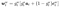the allocation gate g_t^a∈[0,1] governs the interpolation and the write gate g_t^w∈[0,1] determines how much will be written to the memory.

The mechanisms we discussed so far stores no information about the order in which the memory locations are written to. However, there are many situations in which retaining this information is useful: for example when a sequence of instructions must be recorded and retrieved in order. Graves&Wayne et al. propose to keep track of consecutively modified memory locations, thereby enabling a DNC to recover sequences in the written order. This is done as follows

1. We first introduce a precedence weighting p_t ∈𝛥_N, where element p_t[i] represents the degree to which location i was the last one written to. We define p_t by the recurrence relation:

Notice that p_t is updated based on the write weighting w_t^w at the current time step: If 𝛴_i w_t^w[i]≈ 0, which means there is barely any writing happened at the current time step, then p_tp_{t-1}, indicating that the write history is carried over. On the other hand, if 𝛴_iw_t^w[i]≈1, the previous precedence is nearly replaced, so p_tw_t^w.

2. Now we define the temporal link matrix L_t, where L_t[i, j] represents the degree to which location i was written to after location j was written to(one could regard it as a variant of an adjacent matrix). Every time a location is modified, we want to update the link matrix to remove old links to and from that location and add new links from the last-written location. This is done by the following recurrence relation

We exclude self-links since it is unclear how to follow a transition from a location to itself. Noticeably, we use the precedence weighting from the previous time step in the above update, which is sensible because of the definition of the link matrix L_t. We can vectorize the above process as follows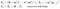E is a matrix of ones and I is an identity matrix.

Before we continue, let’s take some time to rationalize (1-w_t^w[i]-w_t^w[j]. -w_t^w[i] says that the more we write to memory slot i, the less we retain the corresponding link entry. Especially, when w_t^w[i]=1, we retain no more about L_{t-1}[i, j] and our new L_t[i, j] completely depends on w_t^w[i]p_{t-1}[j]. The rationality of w_t^w[j] works in a similar way: The more memory slot j is written to, the newer memory at location j is and the less the corresponding link entry should be retained. When w_t^w[j]=1, i.e., memory at location j is completely refreshed by the new write vector, all L_t[•, j] are reset.

3. We now designate the forward weighting f_t^i∈𝛥_N and backward weighting b_t^i∈𝛥_N for reading head i based on the temporal link matrix

Intuitively, the forward weighting specifies what the head is going to read after the previous reading in the sequential written order, and the backward weighting specifies that in the inverse written order.

Also, Graves&Wayne et al. propose a trick to reduce the overhead of the link matrix. I omit it since I’m not so sure if it worths the effort for that we can vectorize the update.

Each read head leverages a read mode vector 𝜋_t^i∈S_3 to weight the backward weighting b_t^i, the forward weighting f_t^i and the content read weighting c_t^{r,i}=C(M_{t}, k_t^{r,i},\beta_t^{r,i}) as follows

# End

That’s it. It was a long journey; hopefully, you were enjoying it. If you bump into some mistakes or have some concerns, welcome to leave a note/comment.

# References

Alex Graves, Greg Wayne, Malcolm Reynolds, Tim Harley, Ivo Danihelka, Agnieszka Grabska-Barwińska, Sergio Gómez Colmenarejo, Edward Grefenstette, Tiago Ramalho, John Agapiou, Adrià Puigdomènech Badia, Karl Moritz Hermann, Yori Zwols, Georg Ostrovski, Adam Cain, Helen King, Christopher Summerfield, Phil Blunsom, Koray Kavukcuoglu & Demis Hassabis. 2016. “Hybrid Computing Using a Neural Network with Dynamic External Memory.” *Nature* 538 (7626): 471–76. https://doi.org/10.1038/nature20101.

Hsin, Carol. 2016. “Implementation and Optimization of Differentiable Neural Computers.” https://web.stanford.edu/class/cs224n/reports/2753780.pdf.

Written by

## Towards AI

#### Towards AI, is the world’s fastest-growing AI community for learning, programming, building and implementing AI.

Welcome to a place where words matter. On Medium, smart voices and original ideas take center stage - with no ads in sight. Watch
Follow all the topics you care about, and we’ll deliver the best stories for you to your homepage and inbox. Explore
Get unlimited access to the best stories on Medium — and support writers while you’re at it. Just \$5/month. Upgrade